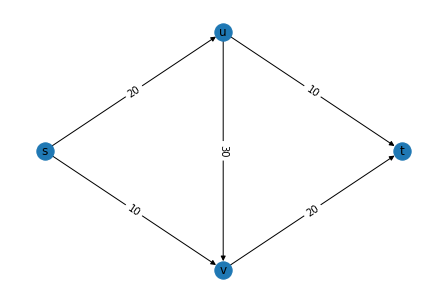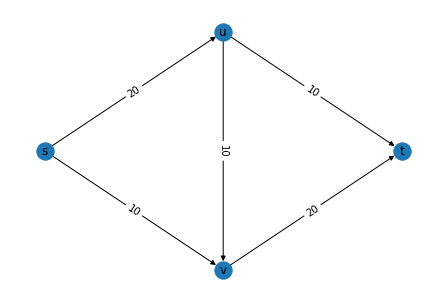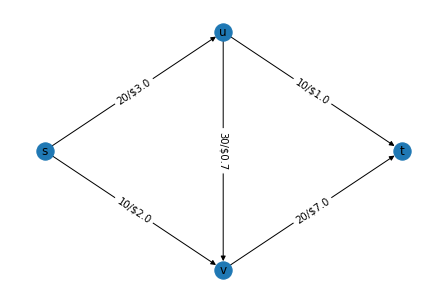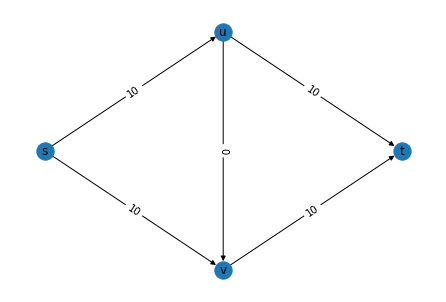# Flow maximisation problems¶

Author: Achyuthuni Sri Harsha
A directed graph has the capacities on all the edges and our job is to find the maximum amount of flow that can happen from one node (starting node) to another node(outflow node). There are two types of flow maximisation problems:
1. Max flow: What is the maximum flow that can be sent from source to output without exceeding capacity.
2. Min cost flow: We have the cost along with capacities on each edge. We want to find the minimum cost path of sending f uits of flow from source to outflow.

We can use integer programming to solve both these problems.

import networkx as nx

# Other packages for manupulating data
import numpy as np
import pandas as pd

# packages for plotting
import matplotlib.pyplot as plt


## Maximum flow problem¶

Let us first consider the max flow problem. Consider the below graph.

edgelist_df = pd.DataFrame({'node1':['s', 's', 'u', 'u', 'v'], 'node2':['u', 'v', 'v', 't', 't'],
'weights':[20, 10, 30, 10, 20]
})
edgelist_df

node1 node2 weights
0 s u 20
1 s v 10
2 u v 30
3 u t 10
4 v t 20
g = nx.DiGraph()
for i, elrow in edgelist_df.iterrows():
g.edges(data=True)

OutEdgeDataView([('s', 'u', {'weight': 20}), ('s', 'v', {'weight': 10}), ('u', 'v', {'weight': 30}), ('u', 't', {'weight': 10}), ('v', 't', {'weight': 20})])

# for each node we are trying to fix the coordinates
g.nodes(data=True)

NodeDataView({'s': {'pos': (0, 1)}, 'u': {'pos': (1, 2)}, 'v': {'pos': (1, 0)}, 't': {'pos': (2, 1)}})

pos = nx.get_node_attributes(g,'pos')

weight = nx.get_edge_attributes(g,'weight')

nx.draw(g,pos, with_labels=True)

nx.draw_networkx_edge_labels(g,pos,edge_labels = weight)
plt.show()We want to find the maximum flow between s and t. This can be formulated as an integer programming problem, with
Decision variable 1. Integer variable $edge_{i,j}$ representing the amount of flow in edge ij. 2. Integer variable f representing the maximum flow

from ortools.sat.python import cp_model
max_flow_model = cp_model.CpModel()

# Creating one integer decision variable for each edge
edge_int_vars = {}
for edge in g.edges:
edge_int_vars[edge, edge] = max_flow_model.NewIntVar(0, 100, 'edge_%s_%s' % edge)
print('Creating the integer variable ', edge_int_vars[edge, edge],
'representing the amount to flow in edge', (edge, edge))

Creating the integer variable  edge_s_u representing the amount to flow in edge ('s', 'u')
Creating the integer variable  edge_s_v representing the amount to flow in edge ('s', 'v')
Creating the integer variable  edge_u_v representing the amount to flow in edge ('u', 'v')
Creating the integer variable  edge_u_t representing the amount to flow in edge ('u', 't')
Creating the integer variable  edge_v_t representing the amount to flow in edge ('v', 't')


Constraints
The first set of constraints are flow constraints, 1. At the input node, the net flow should be -f 2. At the output node, the net flow should be +f 3. The net flow in all other nodes should be 0

input_node = 's'
output_node = 't'

# Adding constraints on the nodes
flow = max_flow_model.NewIntVar(0, 100, 'flow') # Initialising flow this will be maximised later
for node in g.nodes:
in_edges = g.in_edges(node)
out_edges = g.out_edges(node)
print('Adding the constraint on node ', node)
equation_at_this_edge = sum(edge_int_vars[edge, edge] for edge in in_edges) - \
sum(edge_int_vars[edge, edge] for edge in out_edges)
if(node == input_node):
print(equation_at_this_edge == -flow)
elif(node == output_node):
print(equation_at_this_edge == flow)
else:
print(equation_at_this_edge, '== 0')
print('')

Adding the constraint on node  s
((-((edge_s_u) + edge_s_v)) + (1 * flow)) == 0

Adding the constraint on node  u
((edge_s_u) + -((edge_u_v) + edge_u_t)) == 0

Adding the constraint on node  v
(((edge_s_v) + edge_u_v) + -(edge_v_t)) == 0

Adding the constraint on node  t
((((edge_u_t) + edge_v_t)) + -flow) == 0


Another set of constraints are the capacity restrictions on every edge.

# Adding constraints on the edges
for edge in g.edges:
print('Adding constraint on edge ', edge)
max_flow_in_edge = g.get_edge_data(*edge)['weight']
print(edge_int_vars[edge, edge] <= max_flow_in_edge)

Adding constraint on edge  ('s', 'u')
edge_s_u <= 20
Adding constraint on edge  ('s', 'v')
edge_s_v <= 10
Adding constraint on edge  ('u', 'v')
edge_u_v <= 30
Adding constraint on edge  ('u', 't')
edge_u_t <= 10
Adding constraint on edge  ('v', 't')
edge_v_t <= 20


Objective
The objective is to maximise flow.

# The objective is to maximise flow
max_flow_model.Maximize(flow)


Solving the problem, we get

# Solving the problem
solver = cp_model.CpSolver()
solution_printer = cp_model.ObjectiveSolutionPrinter()
status = solver.SolveWithSolutionCallback(max_flow_model, solution_printer)

Solution 0, time = 0.44 s, objective = 30

cp_model.OPTIMAL == status

True

result_edges = {}
for edge in g.edges:
result_edges[edge, edge] = solver.Value(edge_int_vars[edge, edge])
result_edges

{('s', 'u'): 20,
('s', 'v'): 10,
('u', 'v'): 10,
('u', 't'): 10,
('v', 't'): 20}


The flow in each of the nodes is shown in the figure

pos = nx.get_node_attributes(g,'pos')
nx.draw(g,pos, with_labels=True)
nx.draw_networkx_edge_labels(g,pos,edge_labels = result_edges)
plt.show()## Minimum cost flow problems¶

We want to find the minimum cost to transfer a fixed amount of flow from one edge to another. The costs are given in the table below. The costs are also displayed in the network below.

edgelist_df = pd.DataFrame({'node1':['s', 's', 'u', 'u', 'v'], 'node2':['u', 'v', 'v', 't', 't'],
'weights':[20, 10, 30, 10, 20], 'costs':[3, 2, 0.7, 1, 7]
})
edgelist_df

node1 node2 weights costs
0 s u 20 3.0
1 s v 10 2.0
2 u v 30 0.7
3 u t 10 1.0
4 v t 20 7.0
g = nx.DiGraph()
for i, elrow in edgelist_df.iterrows():
g.add_edge(elrow, elrow, weight=elrow, cost = elrow)
g.edges(data=True)

OutEdgeDataView([('s', 'u', {'weight': 20, 'cost': 3.0}), ('s', 'v', {'weight': 10, 'cost': 2.0}), ('u', 'v', {'weight': 30, 'cost': 0.7}), ('u', 't', {'weight': 10, 'cost': 1.0}), ('v', 't', {'weight': 20, 'cost': 7.0})])

# for each node we are trying to fix the coordinates
g.nodes(data=True)

NodeDataView({'s': {'pos': (0, 1)}, 'u': {'pos': (1, 2)}, 'v': {'pos': (1, 0)}, 't': {'pos': (2, 1)}})

pos = nx.get_node_attributes(g,'pos')

weight = nx.get_edge_attributes(g,'weight')
cost = nx.get_edge_attributes(g,'cost')
res = {key: str(weight[key]) + '/\$' + str(cost.get(key, '')) for key in cost.keys()}

nx.draw(g,pos, with_labels=True)

nx.draw_networkx_edge_labels(g,pos,edge_labels = res)
plt.show()Just like maximum flow, we can use integer programming to find out the solution in this scenario also.
Decision variables
Integer variables $edge_{i,j}$ representing the amount of flow in edge (i,j)
Constraints
Capacity restrictions on every edge
Objective The objective is to minimise the overall cost

from ortools.sat.python import cp_model
min_cost_flow_model = cp_model.CpModel()

# Creating one integer decision variable for each edge
edge_int_vars = {}
for edge in g.edges:
edge_int_vars[edge, edge] = min_cost_flow_model.NewIntVar(0, 100, 'edge_%s_%s' % edge)
print('Creating the integer variable ', edge_int_vars[edge, edge],
'representing the amount to flow in edge', (edge, edge))

Creating the integer variable  edge_s_u representing the amount to flow in edge ('s', 'u')
Creating the integer variable  edge_s_v representing the amount to flow in edge ('s', 'v')
Creating the integer variable  edge_u_v representing the amount to flow in edge ('u', 'v')
Creating the integer variable  edge_u_t representing the amount to flow in edge ('u', 't')
Creating the integer variable  edge_v_t representing the amount to flow in edge ('v', 't')

input_node = 's'
output_node = 't'
flow = 20 # Assuming a constant flow of 20 units

# Adding constraints on the nodes
for node in g.nodes:
in_edges = g.in_edges(node)
out_edges = g.out_edges(node)
print('Adding the constraint on node ', node)
print('This node has %i in-edges and %i out-edges' % (len(in_edges), len(out_edges)))
equation_at_this_edge = sum(edge_int_vars[edge, edge] for edge in in_edges) - \
sum(edge_int_vars[edge, edge] for edge in out_edges)
if(node == input_node):
print(equation_at_this_edge == -flow)
elif(node == output_node):
print(equation_at_this_edge == flow)
else:
print(equation_at_this_edge, '== 0')
print('')

Adding the constraint on node  s
This node has 0 in-edges and 2 out-edges
(-((edge_s_u) + edge_s_v)) == -20

Adding the constraint on node  u
This node has 1 in-edges and 2 out-edges
((edge_s_u) + -((edge_u_v) + edge_u_t)) == 0

Adding the constraint on node  v
This node has 2 in-edges and 1 out-edges
(((edge_s_v) + edge_u_v) + -(edge_v_t)) == 0

Adding the constraint on node  t
This node has 2 in-edges and 0 out-edges
(((edge_u_t) + edge_v_t)) == 20

# Adding constraints on the edges
for edge in g.edges:
print('Adding constraint on edge ', edge)
max_flow_in_edge = g.get_edge_data(*edge)['weight']
print(edge_int_vars[edge, edge] <= max_flow_in_edge)

Adding constraint on edge  ('s', 'u')
edge_s_u <= 20
Adding constraint on edge  ('s', 'v')
edge_s_v <= 10
Adding constraint on edge  ('u', 'v')
edge_u_v <= 30
Adding constraint on edge  ('u', 't')
edge_u_t <= 10
Adding constraint on edge  ('v', 't')
edge_v_t <= 20

# factor to make everything including costs integer
factor_to_int = 10

# The objective is to maximise flow
total_cost = sum(int(g.get_edge_data(*edge)['cost']*factor_to_int)*edge_int_vars[edge, edge]
for edge in g.edges)

print('Objective is to optimise cost')
print(total_cost)
min_cost_flow_model.Minimize(total_cost)

Objective is to optimise cost
((((((30 * edge_s_u)) + (20 * edge_s_v)) + (7 * edge_u_v)) + (10 * edge_u_t)) + (70 * edge_v_t))

# Solving the problem
solver = cp_model.CpSolver()
solution_printer = cp_model.ObjectiveSolutionPrinter()
status = solver.SolveWithSolutionCallback(min_cost_flow_model, solution_printer)

Solution 0, time = 0.24 s, objective = 1300

cp_model.OPTIMAL == status

True


The solution to the minimum flow problem is

result_edges = {}
for edge in g.edges:
result_edges[edge, edge] = solver.Value(edge_int_vars[edge, edge])
result_edges

{('s', 'u'): 10, ('s', 'v'): 10, ('u', 'v'): 0, ('u', 't'): 10, ('v', 't'): 10}

# This function gets the coordinates for nodes
pos = nx.get_node_attributes(g,'pos')

# The simplest way to draw a graph is by using nx.draw.
nx.draw(g,pos, with_labels=True)